ON INVERSE FUNCTIONS

With No Domain RestrictionsYou can always find the inverse of a one-to-one function without restricting the domain of the function. Recall that a function is a rule that links an element in the domain to just one number in the range. You could have points (3, 7), (8, 7) and (14,7) on the graph of a function. A one-to-one function adds the requirement that each element in the range is linked to just one number in the domain. In this case, the above three points would not be points on the graph of a one-to-one function because 7 links to different numbers in the domain.

You can identify a one-to-one function from its graph by using the Horizontal Line Test. Mentally scan the graph with a horizontal line; if the line intersects the graph in more than one place, it is not the graph of a one-to-one function. If the function is not one-to-one, then its inverse will not be unique. Therefore, the domain of the original function must be restricted so that the inverse will be unique.

The following is an example of finding the inverse of a one-to-one function.

Example 1: Find the inverse of the function defined as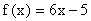Solution:

• If this function has an inverse, we know that the composition of the function and its inverse equals x or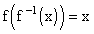. Recall that the original rule takes us from x to f(x) and the inverse rule takes us from f(x) back to x.
• The equationindicates that we use the rule associated with f(x) with the argument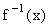.
• The equationcan be written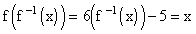or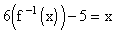.
• Solve forby isolating the term.
• Add 5 to both sides of the equation.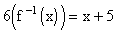• Divide both sides of the equation by 6.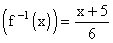.
• The inverse function is the function.
• Let’s check the inverse with a few points. The original functionlinks 2 to 7 because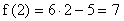. Let’s check to see if the inverse function will indeed link the 7 back to the 2.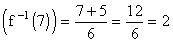. It does.
• You can also graph the original function, the inverse function, and the line y = x. If you fold the graph paper over the line y = x, the graphs of the original function and the inverse function will be superimposed.

Check the graphs out below. Notice also that for every point (a, b) on the graph of the original function, you can find the point (b, a) on the graph of the inverse function.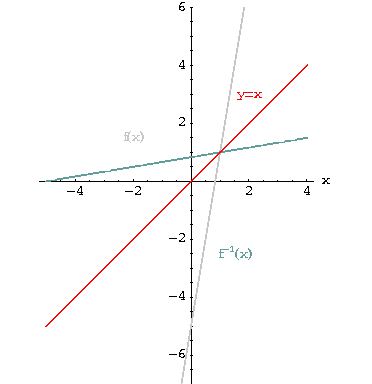Another example of finding the inverse of a function where the domain of the original function does not need to be restricted.[Back] [Next]
[Algebra] [Trigonometry] [Complex Variables]
[Calculus] [Differential Equations] [Matrix Algebra]S.O.S MATHematics home page

Do you need more help? Please post your question on our S.O.S. Mathematics CyberBoard.Author:Nancy Marcus